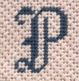Designs by Petei
The Line
The Line List contains the following:
• Design number
• Brief description of the design
• Approximate size of the canvas or congress cloth each design is painted on
• Canvas or congress cloth color, if it is other than white.
All listings contain thumbnail images.

All Designs by Petei are stitch-painted by hand on 18 count mono Zwiegart canvas, except for the designs on congress cloth which are approximately 23 to 24 stitches per inch.

Proceed to The Line - Contents by Category

Designs by Petei hold the Copyrights

on all the designs and books listed.

Square Inch Size Legend

 9 = 3 x 3 20 = 5 x 4 20¼ = 4½ x 4½ 30 = 5 x 6 30¼ = 5½ x 5½ 35 = 5 x 7 40 = 5 x 8 42¼ = 6½ x 6½ 45 = 5 x 9 48 = 6 x 8 54 = 6 x 9 56¼ = 7½ x 7½ 60 = 5 x 12 63 = 7 x 9 64 = 8 x 8 68 = 8 x 8½ 81 = 9 x 9 100 = 10 x 10 120 = 12 x 10 130 = 10 x 13 132 = 11 x 12 150 = 10 x 15 156 = 12 x 13 175 = 17½ x 10 201¼ = 17½ x 11½

All canvas sizes indicated are in inches and are approximate.

Size = Width x Height (Square Inches).

There are no suggested retail prices on this list.

Images may not be shown to scale and only show design area.

All current design images are shown.

Some designs may be listed in more than one category.

Links from design listings are to stitched samples.

Proceed to The Line - Contents by Category

Proceed to the Terms & Conditions